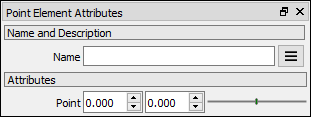# Point Element node

Left Toolbar >Math Nodes >Point Element

The Point Element node output separate point x and y values of the input point.

## Procedure

### To define a point element in the node graph:

1. On the left toolbar choose Point Nodes> Element Point2. Drag the Point Element icon into the Node Graph.3. Connect the Output: x or y.

## Inputs

point

Point Value (x,y).

## Outputs

X

X position. A NUMBER type of value.

Y

Y position. A NUMBER type of value.

## Attributes PanelPoint

Point Value. This attribute is exposed as input by default. Right-click the Node to hide this attribute as input and set the point values manually.

Math Nodes

Main Page